# Quantitative MCQ - 29

## 30 Questions MCQ Test Quantitative Aptitude for Competitive Examinations | Quantitative MCQ - 29

Description
Attempt Quantitative MCQ - 29 | 30 questions in 30 minutes | Mock test for Quant preparation | Free important questions MCQ to study Quantitative Aptitude for Competitive Examinations for Quant Exam | Download free PDF with solutions
QUESTION: 1

### Directions (1-5): What should come in place of question mark (?) in the following number series?  567, 295, 159, 91, 57, ?

Solution: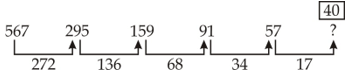QUESTION: 2

### Directions (1-5): What should come in place of question mark (?) in the following number series? 76, 115, 64, 103, 52, ?

Solution: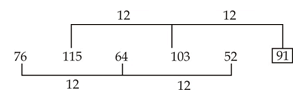QUESTION: 3

### Directions (1-5): What should come in place of question mark (?) in the following number series? 963, 927, 855, 747, 603, 423, ?

Solution: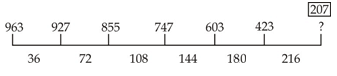QUESTION: 4

Directions (1-5): What should come in place of question mark (?) in the following number series?

23, 15, 22, 58, 224, ?

Solution: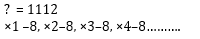QUESTION: 5

Directions (1-5): What should come in place of question mark (?) in the following number series?

7, 42, 504, ?, 217728, 6531840

Solution: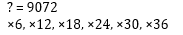QUESTION: 6

Directions (6-10): In the following questions two equations numbered (I) and (II) are given. You have to solve both equations and give answer

I. x² + 15x + 56 = 0

II. y² + 17y + 72 = 0

Solution: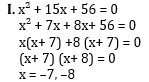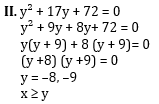QUESTION: 7

Directions (6-10): In the following questions two equations numbered (I) and (II) are given. You have to solve both equations and give answer

I. 2x² – 9x + 10 = 0

II. 9y² - 57y + 88 = 0

Solution: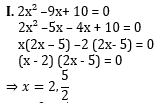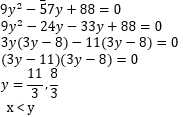QUESTION: 8

Directions (6-10): In the following questions two equations numbered (I) and (II) are given. You have to solve both equations and give answer

I. 3x + 2y = 7√14

II. 10x + 14y =38√14

Solution: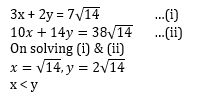QUESTION: 9

Directions (6-10): In the following questions two equations numbered (I) and (II) are given. You have to solve both equations and give answer

I. x² - 11x + 28 = 0

II. y² + 3y – 40 = 0

Solution: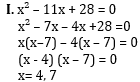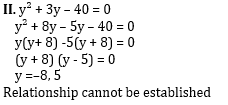QUESTION: 10

Directions (6-10): In the following questions two equations numbered (I) and (II) are given. You have to solve both equations and give answer

I. x² = 361

II. y = √441

Solution: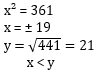QUESTION: 11

Directions (Q.11 - 15) : What will come in place of question mark (?) in the following number series?

26, 12, 10, 16, ?

Solution: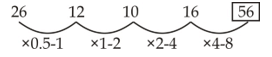QUESTION: 12

Directions (Q.11 - 15) : What will come in place of question mark (?) in the following number series?

82, 107, 207, 432, 832, ?

Solution: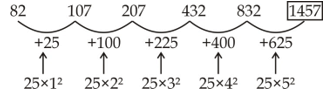QUESTION: 13

Directions (Q.11 - 15) : What will come in place of question mark (?) in the following number series?

122, 213, 340, 509, ?, 997

Solution: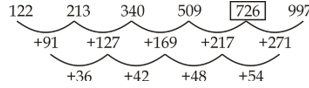QUESTION: 14

Directions (Q.11 - 15) : What will come in place of question mark (?) in the following number series?

25, 37.5, 56.25, ?, 126.5625

Solution: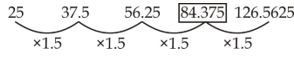QUESTION: 15

Directions (Q.11 - 15) : What will come in place of question mark (?) in the following number series?

10, 50, 94, 142, 194, ?

Solution: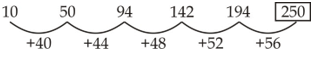QUESTION: 16

In a mixture of milk and water the proportion of water by weight was 75%. If in the 60 gms mixture 15 gms water was added, what would be the percentage of water in the new mixture?

Solution: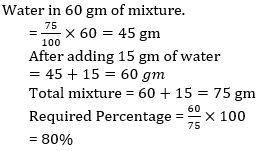QUESTION: 17

If 36 persons are engaged on a piece of work, the work can be completed in 40 days. After 32 days, only 3/4th of the work was completed. How many more persons are required to complete the work on time?

Solution: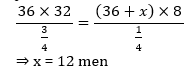QUESTION: 18

The simple interest accrued on an amount of Rs. 22,500 at the end of four years is Rs. 10,800. What would be the compound interest accrued on the same amount at the same rate at the end of two years?

Solution: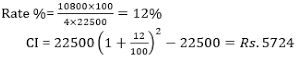QUESTION: 19

If the length of a rectangular field is increased by 20% and the breadth is reduced by 20%, the area of the rectangle will be 192m2. What is the area of the original rectangle?

Solution: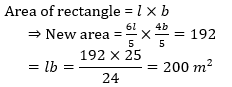QUESTION: 20

Directions (20-24): What should come in place of question mark (?) in the following number series?

1, 1, 3, 9, 13, 65, 71, ?, 505

Solution: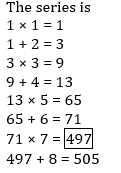QUESTION: 21

Directions (20-24): What should come in place of question mark (?) in the following number series?

1, 2, 6, 42, ?, 3263442

Solution: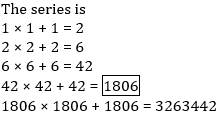QUESTION: 22

Directions (20-24): What should come in place of question mark (?) in the following number series?

56, 57, 48, 73, 24, 105, ?

Solution: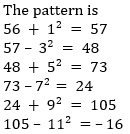QUESTION: 23

Directions (20-24): What should come in place of question mark (?) in the following number series?

3, 1, 3, 0.75, 3, 0.6, 3, ?

Solution: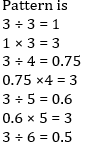QUESTION: 24

Directions (20-24): What should come in place of question mark (?) in the following number series?

2, 5, 10, 17, 28, 41, 58, ?

Solution: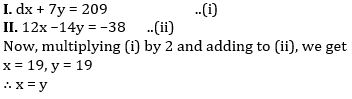QUESTION: 25

Directions (25-29): In the following questions two equations numbered I and II are given. Solve the equation and answer the question if:

I. 4x + 7y = 209
II. 12x – 14y = -38

Solution: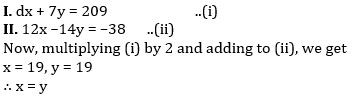QUESTION: 26

Directions (25-29): In the following questions two equations numbered I and II are given. Solve the equation and answer the question if:

I. (17)2 + 144 ÷ 18 = x
II. (26)2 – 18 × 21 = y

Solution: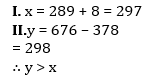QUESTION: 27

Directions (25-29): In the following questions two equations numbered I and II are given. Solve the equation and answer the question if:

I. x2– 11x + 24 = 0
II. 2y2 – 9y + 9 = 0

Solution: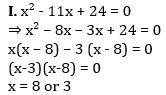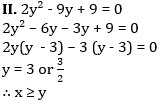QUESTION: 28

Directions (25-29): In the following questions two equations numbered I and II are given. Solve the equation and answer the question if:

I. 18x2 + 18x + 4 = 0
II. 12y2 + 29y + 14 = 0

Solution: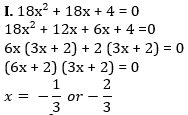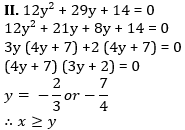QUESTION: 29

Directions (25-29): In the following questions two equations numbered I and II are given. Solve the equation and answer the question if:

I. 8x2 + 6x = 5
II. 12y2 – 22y + 8 = 0

Solution: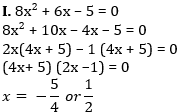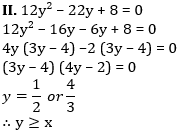QUESTION: 30

What is the ratio of the total number of votes polled in Chandani Chowk and Purani Delhi together in 2008 to the total number of votes polled in these two constituencies together in 2013 ?

Solution: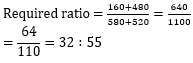Use Code STAYHOME200 and get INR 200 additional OFF Use Coupon Code